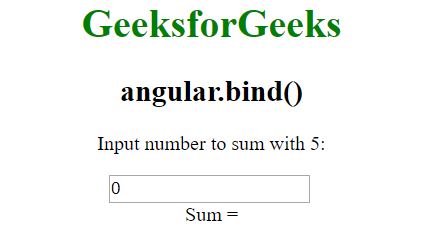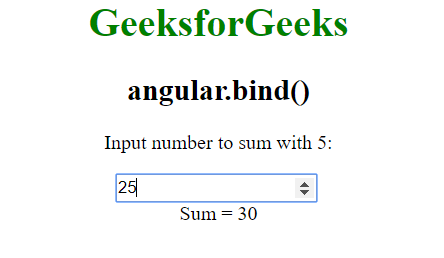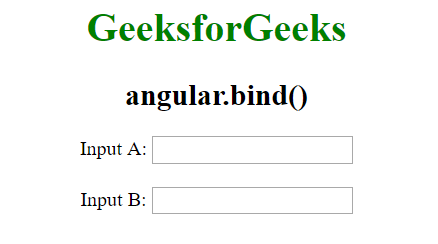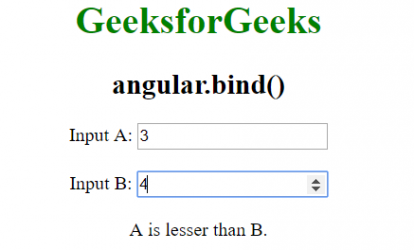Related Articles

# AngularJS | angular.bind() Function

• Last Updated : 30 Mar, 2021

The angular.bind() Function in AngularJS is used to bind the current context to a function, but actually execute it at a later time. It can also be used in Partial Applications. Partial Applications is when you want to make a function but some of the arguments have been passed already.
Syntax:

`angular.bind(self, function, args);`

Parameter Values:

• self: This refers to the context in which the function should be evaluated.
• function: It refers to the function to be bound.
• args: It is used to prebound to the function at the time of function call. It is an optional argument.

Example 1:

## html

 `<``html``>``   ``<``head``>``      ``<``title``>angular.bind()``      ``<``script` `src``=``         ``"https://ajax.googleapis.com/ajax/libs/angularjs/1.5.6/angular.min.js"``>``      ````   ``` `   ``<``body` `ng-app``=``"app"` `style``=``"text-align:Center"``>` `      ``<``h1` `style``=``"color:green"``>GeeksforGeeks` `      ``<``h2``>angular.bind()` `      ` `<``p``>Input number to sum with 5:``      ``<``div` `ng-controller``=``"geek"``>``         ``<``input` `type``=``"number"` `ng-model``=``"num"` `ng-change``=``"Func()"` `/>``         ``<``br``>Sum = {{Add}}``      ``` `      ``<``script``>``         ``var app = angular.module("app", []);``         ``app.controller('geek', ['\$scope', function (\$scope) {``             ``\$scope.num = 0;``             ``\$scope.Func = function () {``                 ``var add = angular.bind(this, function (a, b) {``                     ``return a + b;``                 ``});``                 ``\$scope.Add = add(5, \$scope.num);``             ``}``         ``}]);``         ` `      ````   `````

Output:
Before Input:After Input:Example 2:

## html

 `<``html``>``   ``<``head``>``      ``<``title``>angular.bind()``      ``<``script` `src``=``          ``"https://ajax.googleapis.com/ajax/libs/angularjs/1.5.6/angular.min.js"``>``      ````   ``` `   ``<``body` `ng-app``=``"app"` `style``=``"text-align:Center"``>` `      ``<``h1` `style``=``"color:green"``>GeeksforGeeks` `      ``<``h2``>angular.bind()` `      ``<``div` `ng-controller``=``"geek"``>``         ``Input A:``         ``<``input` `type``=``"number"` `ng-model``=``"val1"` `ng-change``=``"GetResult()"` `/>``         ``<``br``><``br``>``         ``Input B:``         ``<``input` `type``=``"number"` `ng-model``=``"val2"` `ng-change``=``"GetResult()"` `/>``         ``<``br` `/><``br``>``         ``{{result}}``      ``` `      ``<``script``>``         ``var app = angular.module("app", []);``         ``app.controller('geek', ['\$scope', function (\$scope) {``             ``function isEqual(a, b) {``                 ``if (a == b) {``                     ``return "Inputs are equal."``                 ``}``                 ``else if (a >= b) {``                     ``return "A is greater than B."``                 ``}``                 ``else if (a <= b) {``                     ``return "A is lesser than B."``                 ``}``             ``}``             ``\$scope.GetResult = function () {``                 ``var result = angular.bind(this, isEqual);``                 ``\$scope.result = result(\$scope.val1, \$scope.val2);``             ``}``         ``}]);``         ` `      ````   `````

Output:
Before Input:After Input:My Personal Notes arrow_drop_up